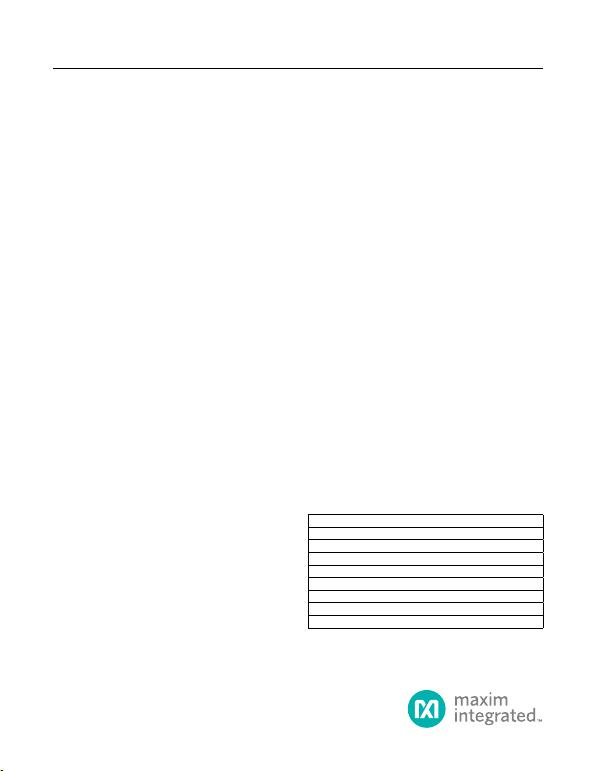General Description
The MAX15004A/B/MAX15005A/B high-performance,
current-mode PWM controllers operate at an automotive
input voltage range from 4.5V to 40V (load dump). The
input voltage can go lower than 4.5V after startup if IN is
bootstrapped to a boosted output voltage. The controllers
integrate all the building blocks necessary for implement-
ing fixed-frequency isolated/nonisolated power supplies.
The general-purpose boost, flyback, forward, and SEPIC
converters can be designed with ease around the
MAX15004/MAX15005.
The current-mode control architecture offers excellent line-
transient response and cycle-by-cycle current limit while
simplifying the frequency compensation. Programmable
slope compensation simplifies the design further. A fast
60ns current-limit response time, low 300mV current-limit
threshold makes the controllers suitable for high-efficiency,
high-frequency DC-DC converters. The devices include
an internal error amplifier and 1% accurate reference to
facilitate the primary-side regulated, single-ended flyback
converter or nonisolated converters.
An external resistor and capacitor network programs the
switching frequency from 15kHz to 500kHz (1MHz for
the MAX15005A/B). The MAX15004A/B/MAX15005A/B
provide a SYNC input for synchronization to an
external clock. The maximum FET-driver duty cycle for the
MAX15004A/B is 50%. The maximum duty cycle can be
set on the MAX15005A/B by selecting the right combina-
tion of RT and CT.
The input undervoltage lockout (ON/OFF) programs the
input-supply startup voltage and can be used to shutdown
the converter to reduce the total shutdown current down
to 10µA. Protection features include cycle-by-cycle and
hiccup current limit, output overvoltage protection, and
thermal shutdown.
The MAX15004A/B/MAX15005A/B are available in space-
saving 16-pin TSSOP and thermally enhanced 16-pin
TSSOP-EP packages. All devices operate over the -40°C
to +125°C automotive temperature range.
Applications
Automotive
Vacuum Fluorescent Display (VFD) Power Supply
Isolated Flyback, Forward, Nonisolated SEPIC, Boost
Converters
Benets and Features
Wide Supply Voltage Range Meets Automotive
Power-Supply Operating Requirement Including
“Cold Crank” Conditions
4.5V to 40V Operating Input Voltage Range
(Can Operate at Lower Voltage After Startup if
Input is Bootstrapped to a Boosted Output)
Control Architecture Offers Excellent Performance
While Simplifying the Design
Current-Mode Control
300mV, 5% Accurate Current-Limit Threshold
Voltage
Programmable Slope Compensation
Maximum Duty Cycle
Synchronization Avoids Interference with Sensitive
Switching Frequency Adjustable from 15kHz to
500kHz (1MHz for the MAX15005A/B)
RC Programmable 4% Accurate Switching
Frequency
External Frequency Synchronization
Built-In Protection Capability for Improved System
Reliability
Cycle-by-Cycle and Hiccup Current-Limit
Protection
Overvoltage and Thermal-Shutdown Protection
-40°C to +125°C Automotive Temperature Range
AEC-Q100 Qualified
19-0723; Rev 7; 2/17
Pin Configuration appears at end of data sheet.
Note: All devices are specified over the -40°C to +125°C
temperature range.
/V denotes an automotive qualified part.
PART PIN-PACKAGE MAX DUTY CYCLE
MAX15004AAUE+ 16 TSSOP-EP* 50%
MAX15004AAUE/V+ 16 TSSOP-EP* 50%
MAX15004BAUE+ 16 TSSOP 50%
MAX15004BAUE/V+ 16 TSSOP 50%
MAX15005AAUE+ 16 TSSOP-EP* Programmable
MAX15005AAUE/V+ 16 TSSOP-EP* Programmable
MAX15005BAUE+ 16 TSSOP Programmable
MAX15005BAUE/V+ 16 TSSOP Programmable
MAX15004A/B-MAX15005A/B 4.5V to 40V Input Automotive
Flyback/Boost/SEPIC
Power-Supply Controllers
Ordering Information
EVALUATION KIT AVAILABLE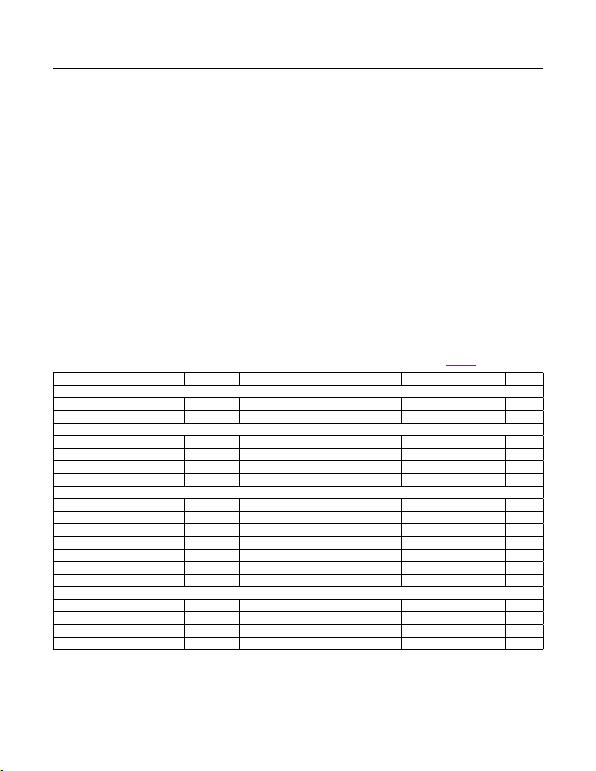IN to SGND ...........................................................-0.3V to +45V
IN to PGND ...........................................................-0.3V to +45V
ON/OFF to SGND ......................................-0.3V to (VIN + 0.3V)
OVI, SLOPE, RTCT, SYNC, SS, FB, COMP,
CS to SGND ..................................... -0.3V to (VREG5 + 0.3V)
VCC to PGND ........................................................-0.3V to +12V
REG5 to SGND .......................................................-0.3V to +6V
OUT to PGND .......................................... -0.3V to (VCC + 0.3V)
SGND to PGND ....................................................-0.3V to +0.3V
VCC Sink Current (clamped mode) ....................................35mA
OUT Current (< 10μs transient) .........................................±1.5A
Continuous Power Dissipation* (TA = +70°C)
16-Pin TSSOP-EP (derate 21.3mW/°C
above +70°C) ............................................................1702mW
16-Pin TSSOP (derate 9.4mW/°C above +70°C) ........ 754mW
Operating Junction Temperature Range .......... -40°C to +125°C
Junction Temperature ...................................................... +150°C
Storage Temperature Range ............................ -60°C to +150°C
Soldering Temperature (reflow) ....................................... +260°C
(VIN = 14V, CIN = 0.1μF, CVCC = 0.1μF // 1μF, CREG5 =F, VON/OFF = 5V, CSS = 0.01μF, CSLOPE = 100pF, RT = 13.7kΩ, CT = 560pF,
VSYNC = VOVI = VFB = VCS = 0V, COMP = unconnected, OUT = unconnected. TA = TJ = -40°C to +125°C, unless otherwise noted.
Typical values are at TA = +25°C. All voltages are referenced to PGND, unless otherwise noted.) (Note 1) (Figure 5)
*As per JEDEC51 Standard, Multilayer Board.
PARAMETER SYMBOL CONDITIONS MIN TYP MAX UNITS
POWER SUPPLY
Input Supply Range VIN 4.5 40.0 V
Operating Supply Current IQVIN = 40V, fOSC = 150kHz 2 3.1 mA
ON/OFF CONTROL
Input-Voltage Threshold VON VON/OFF rising 1.05 1.23 1.40 V
Input-Voltage Hysteresis VHYST-ON 75 mV
Input Bias Current IB-ON/OFF VON/OFF = 40V 0.5 µA
Shutdown Current ISHDN VON/OFF = 0V 10 20 µA
INTERNAL 7.4V LDO (VCC)
Output (VCC) Voltage Set Point VVCC IVCC = 0 to 20mA (sourcing) 7.15 7.4 7.60 V
Line Regulation VIN = 8V to 40V 1 mV/V
UVLO Threshold Voltage V UVLO-VCC VCC rising 3.15 3.5 3.75 V
UVLO Hysteresis VHYST-UVLO 500 mV
Dropout Voltage VIN = 4.5V, IVCC = 20mA (sourcing) 0.25 0.5 V
Output Current Limit IVCC-ILIM IVCC sourcing 45 mA
Internal Clamp Voltage VVCC-CLAMP IVCC = 30mA (sinking) 10.0 10.4 10.8 V
INTERNAL 5V LDO (REG5)
Output (REG5) Voltage Set Point VREG5 VCC = 7.5V, IREG5 = 0 to 15mA (sourcing) 4.75 4.95 5.05 V
Line Regulation VCC = 5.5V to 10V 2 mV/V
Dropout Voltage VCC = 4.5V, IREG5 = 15mA (sourcing) 0.25 0.5 V
Output Current Limit IREG5-ILIM IREG5 sourcing 32 mA
MAX15004A/B-MAX15005A/B 4.5V to 40V Input Automotive
Flyback/Boost/SEPIC
Power-Supply Controllers
www.maximintegrated.com Maxim Integrated
2
Absolute Maximum Ratings
Stresses beyond those listed under “Absolute Maximum Ratings” may cause permanent damage to the device. These are stress ratings only, and functional operation of the device at these
or any other conditions beyond those indicated in the operational sections of the specifications is not implied. Exposure to absolute maximum rating conditions for extended periods may affect
device reliability.
Electrical Characteristics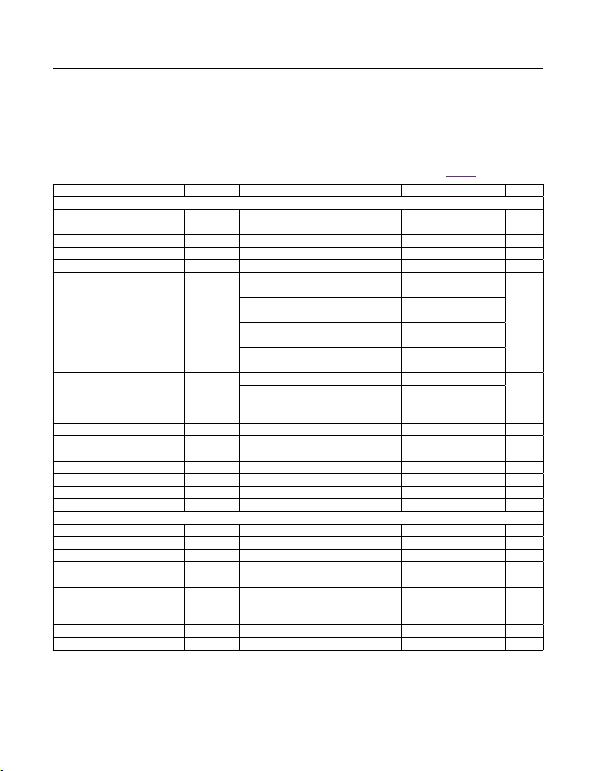(VIN = 14V, CIN = 0.1μF, CVCC = 0.1μF // 1μF, CREG5 =F, VON/OFF = 5V, CSS = 0.01μF, CSLOPE = 100pF, RT = 13.7kΩ, CT = 560pF,
VSYNC = VOVI = VFB = VCS = 0V, COMP = unconnected, OUT = unconnected. TA = TJ = -40°C to +125°C, unless otherwise noted.
Typical values are at TA = +25°C. All voltages are referenced to PGND, unless otherwise noted.) (Note 1) (Figure 5)
PARAMETER SYMBOL CONDITIONS MIN TYP MAX UNITS
OSCILLATOR (RTCT)
Oscillator Frequency Range fOSC
fOSC = 2 x fOUT for MAX15004A/B,
fOSC = fOUT for MAX15005A/B 15 1000 kHz
RTCT Peak Trip Level VTH,RTCT 0.55 x VREG5 V
RTCT Valley Trip Level VTL,RTCT 0.1 x VREG5 V
RTCT Discharge Current IDIS,RTCT VRTCT = 2V 1.30 1.33 1.36 mA
Oscillator Frequency Accuracy
(Note 2)
RT = 13.7kΩ, CT = 4.7nF,
fOSC (typ) = 18kHz -4 +4
%
RT = 13.7kΩ, CT = 560pF,
fOSC (typ) = 150kHz -4 +4
RT = 21kΩ, CT = 100pF,
fOSC (typ) = 500kHz -5 +5
RT = 7kΩ, CT = 100pF,
fOSC (typ) = 1MHz -7 +7
Maximum PWM Duty Cycle
(Note 3) DMAX
MAX15004A/B 50
%
MAX15005A/B,
RT = 13.7kΩ, CT = 560pF,
fOSC (typ) = 150kHz
78.5 80 81.5
Minimum On-Time tON-MIN VIN = 14V 110 170 ns
SYNC Lock-In Frequency Range
(Note 4)
RT = 13.7kΩ, CT = 560pF,
fOSC (typ) = 150kHz 102 200 %fOSC
SYNC High-Level Voltage VIH-SYNC 2 V
SYNC Low-Level Voltage VIL-SYNC 0.8 V
SYNC Input Current ISYNC VSYNC = 0 to 5V -0.5 +0.5 µA
SYNC Minimum Input Pulse Width 50 ns
ERROR AMPLIFIER/SOFT-START
Soft-Start Charging Current ISS VSS = 0V 8 15 21 µA
SS Reference Voltage VSS 1.215 1.228 1.240 V
SS Threshold for HICCUP Enable VSS rising 1.1 V
FB Regulation Voltage VREF-FB
COMP = FB,
ICOMP = -500µA to +500µA 1.215 1.228 1.240 V
FB Input Offset Voltage VOS-FB
COMP = 0.25V to 4.5V,
ICOMP = -500µA to +500µA,
VSS = 0 to 1.5V
-5 +5 mV
FB Input Current VFB = 0 to 1.5V -300 +300 nA
COMP Sink Current ICOMP-SINK VFB = 1.5V, VCOMP = 0.25V 3 5.5 mA
MAX15004A/B-MAX15005A/B 4.5V to 40V Input Automotive
Flyback/Boost/SEPIC
Power-Supply Controllers
www.maximintegrated.com Maxim Integrated
3
Electrical Characteristics (continued)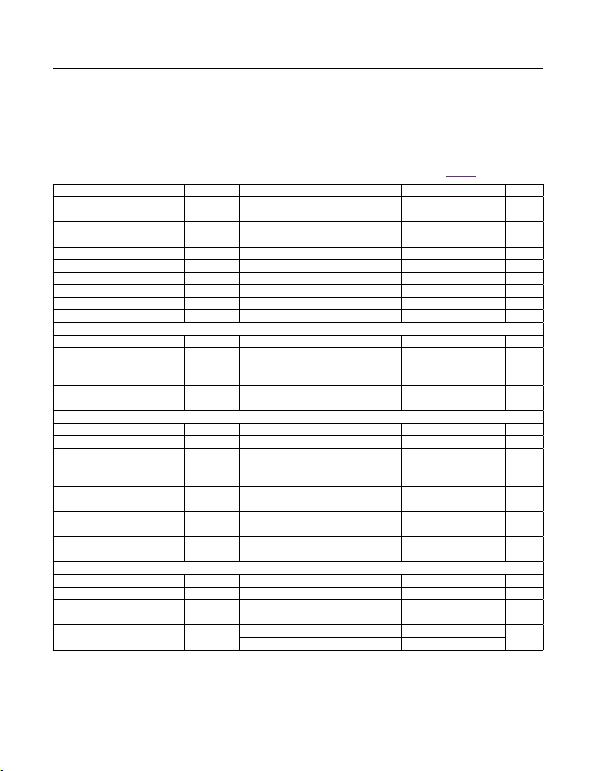(VIN = 14V, CIN = 0.1μF, CVCC = 0.1μF // 1μF, CREG5 =F, VON/OFF = 5V, CSS = 0.01μF, CSLOPE = 100pF, RT = 13.7kΩ, CT = 560pF,
VSYNC = VOVI = VFB = VCS = 0V, COMP = unconnected, OUT = unconnected. TA = TJ = -40°C to +125°C, unless otherwise noted.
Typical values are at TA = +25°C. All voltages are referenced to PGND, unless otherwise noted.) (Note 1) (Figure 5)
PARAMETER SYMBOL CONDITIONS MIN TYP MAX UNITS
COMP Source Current ICOMP-
SOURCE
VFB = 1V, VCOMP = 4.5V 1.3 2.8 mA
COMP High Voltage VOH-COMP VFB = 1V, ICOMP = 1mA (sourcing) VREG5
- 0.5
VREG5
- 0.2 V
COMP Low Voltage VOL-COMP VFB = 1.5V, ICOMP = 1mA (sinking) 0.1 0.25 V
Open-Loop Gain AEAMP 100 dB
Unity-Gain Bandwidth UGFEAMP 1.6 MHz
Phase Margin PMEAMP 75 degrees
COMP Positive Slew Rate SR+ 0.5 V/µs
COMP Negative Slew Rate SR- -0.5 V/µs
PWM COMPARATOR
Current-Sense Gain ACS-PWM ΔVCOMP/ΔVCS (Note 5) 2.85 3 3.15 V/V
PWM Propagation Delay to OUT tPD-PWM
CS = 0.15V, from VCOMP falling edge:
3V to 0.5V to OUT falling (excluding
60 ns
PWM Comparator Current-Sense
Leading-Edge Blanking Time tCS-BLANK 50 ns
CURRENT-LIMIT COMPARATOR
Current-Limit Threshold Voltage VILIM 290 305 317 mV
Current-Limit Input Bias Current IB-CS OUT= high, 0 ≤ VCS ≤ 0.3V -2 +2 µA
ILIMIT Propagation Delay to OUT tPD-ILIM
From CS rising above VILIM (50mV
overdrive) to OUT falling (excluding
60 ns
ILIM Comparator Current-Sense
Leading-Edge Blanking Time tCS-BLANK 50 ns
Number of Consecutive ILIMIT
Events to HICCUP 7
HICCUP Timeout 512 Clock
periods
SLOPE COMPENSATION (Note 6)
Slope Capacitor Charging Current ISLOPE VSLOPE = 100mV 9.8 10.5 11.2 µA
Slope Compensation CSLOPE = 100pF 25 mV/µs
Slope Compensation Tolerance
(Note 2) CSLOPE = 100pF -4 +4 %
Slope Compensation Range CSLOPE = 22pF 110 mV/µs
CSLOPE = 1000pF 2.5
MAX15004A/B-MAX15005A/B 4.5V to 40V Input Automotive
Flyback/Boost/SEPIC
Power-Supply Controllers
www.maximintegrated.com Maxim Integrated
4
Electrical Characteristics (continued)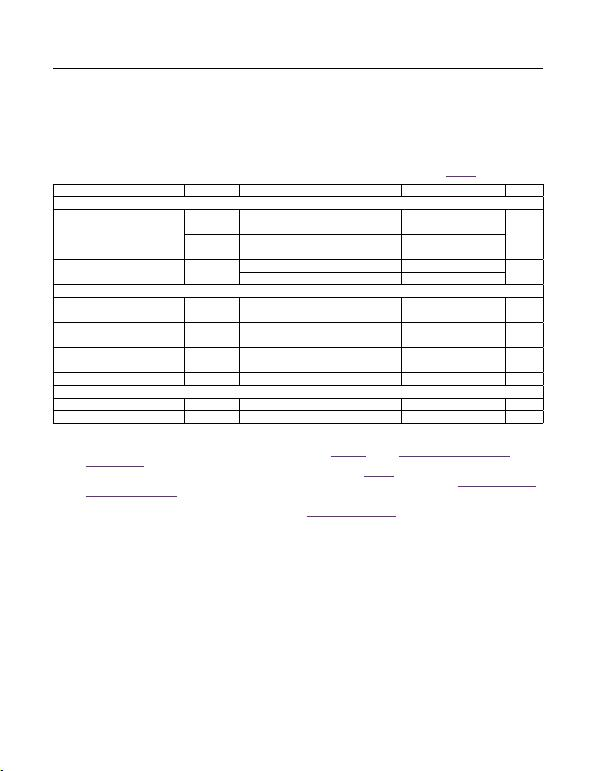(VIN = 14V, CIN = 0.1μF, CVCC = 0.1μF // 1μF, CREG5 =F, VON/OFF = 5V, CSS = 0.01μF, CSLOPE = 100pF, RT = 13.7kΩ, CT = 560pF,
VSYNC = VOVI = VFB = VCS = 0V, COMP = unconnected, OUT = unconnected. TA = TJ = -40°C to +125°C, unless otherwise noted.
Typical values are at TA = +25°C. All voltages are referenced to PGND, unless otherwise noted.) (Note 1) (Figure 5)
Note 1: 100% production tested at +125°C. Limits over the temperature range are guaranteed by design.
Note 2: Guaranteed by design; not production tested.
Note 3: For the MAX15005A/B, DMAX depends upon the value of RT. See Figure 3b and the Oscillator Frequency/External
Synchronization section.
Note 4: The external SYNC pulse triggers the discharge of the oscillator ramp. See Figure 2. During external SYNC, DMAX = 50%
for the MAX15004A/B; for the MAX15005A/B, there is a shift in DMAX with fSYNC/fOSC ratio (see the Oscillator Frequency/
External Synchronization section).
Note 5: The parameter is measured at the trip point of latch, with 0 ≤ VCS 0.3V, and FB = COMP.
Note 6: Slope compensation = (2.5 x 10-9)/CSLOPE mV/μs. See the Applications Information section.
PARAMETER SYMBOL CONDITIONS MIN TYP MAX UNITS
OUTPUT DRIVER
Driver Output Impedance
ROUT-N
VCC = 8V (applied externally),
IOUT = 100mA (sinking) 1.7 3.5
ROUT-P
VCC = 8V (applied externally),
IOUT = 100mA (sourcing) 3 5
Driver Peak Output Current IOUT-PEAK
COUT = 10nF, sinking 1000 mA
COUT = 10nF, sourcing 750
OVERVOLTAGE COMPARATOR
Overvoltage Comparator Input
Threshold VOV-TH VOVI rising 1.20 1.228 1.26 V
Overvoltage Comparator
Hysteresis VOV-HYST 125 mV
Overvoltage Comparator Delay TDOVI
From OVI rising above 1.228V to OUT
falling, with 50mV overdrive 1.6 µs
OVI Input Current IOVI VOVI = 0 to 5V -0.5 +0.5 µA
THERMAL SHUTDOWN
Shutdown Temperature TSHDN Temperature rising 160 °C
Thermal Hysteresis THYST 15 °C
MAX15004A/B-MAX15005A/B 4.5V to 40V Input Automotive
Flyback/Boost/SEPIC
Power-Supply Controllers
www.maximintegrated.com Maxim Integrated
5
Electrical Characteristics (continued)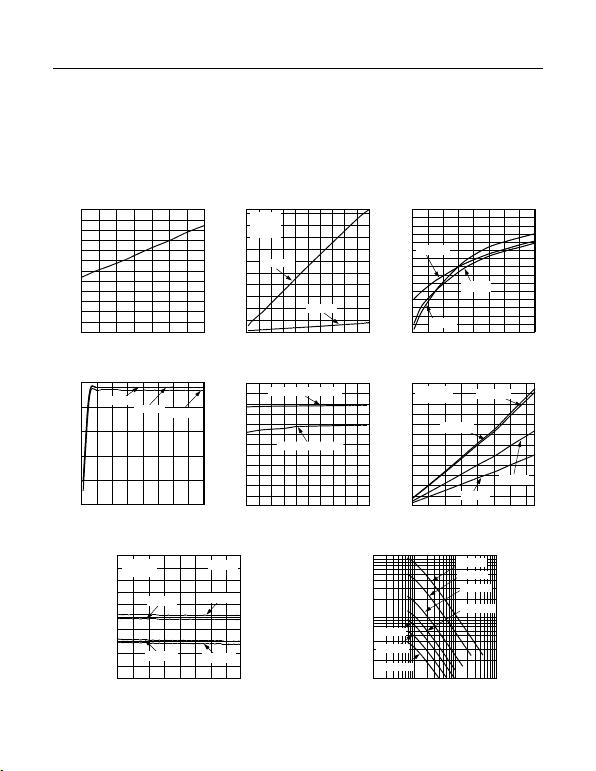VIN = 14V, CIN = 0.1μF, CVCC = 0.1μF // 1μF, CREG5 = 1μF, VON/OFF = 5V, CSS = 0.01μF, CSLOPE = 100pF, RT = 13.7kΩ,
CT = 560pF. TA = +25°C, unless otherwise noted.)
VIN SUPPLY CURRENT (ISUPPLY)
vs. OSCILLATOR FREQUENCY (fOSC)
FREQUENCY (kHz)
V
IN
SUPPLY CURRENT (mA)
MAX15004 toc02
10 60 110 160 210 260 310 360 410 460 510
1
4
7
10
13
16
19
22
25
28
31 MAX15005
VIN = 14V
CT = 220pF
COUT = 10nF
COUT = 0nF
SHUTDOWN SUPPLY CURRENT
vs. SUPPLY VOLTAGE
SUPPLY VOLTAGE (V)
VIN SHUTDOWN SUPPLY CURRENT (µA)
MAX15004 toc03
5 10 15 20 25 30 35 40 45
5
6
7
8
9
10
11
12
13
14
15
16
17
18
19
20
TA = +135°C
TA = -40°C
TA = +25°C
VCC OUTPUT VOLTAGE
vs. VIN SUPPLY VOLTAGE
VIN SUPPLY VOLTAGE (V)
V
CC
OUTPUT VOLTAGE (V)
MAX15004 toc04
5 10 15 20 25 30 35 40 45
5.0
5.5
6.0
6.5
7.0
7.5
IVCC = 0mA
IVCC = 1mA IVCC = 20mA
REG5 OUTPUT VOLTAGE
vs. VCC VOLTAGE
V
CC
VOLTAGE (V)
REG5 OUTPUT VOLTAGE (V)
MAX15004 toc06
5.5 6.0 6.5 7.0 7.5 8.0 8.5 9.0 9.5 10.0 10.5
4.700
4.725
4.750
4.775
4.800
4.825
4.850
4.875
4.900
4.925
4.950
4.975
5.000
IREG5 = 1mA (SOURCING)
IREG5 = 15mA (SOURCING)
REG5 DROPOUT VOLTAGE
vs. IREG5
I
REG5
(mA)
REG5 LDO DROPOUT VOLTAGE (V)
MAX15004 toc07
0 2 4 6 8 10 12 14
0
0.03
0.05
0.08
0.10
0.13
0.15
0.18
0.20
0.23
0.25
0.28
0.30
TA = +135°C
TA = +25°C
TA = -40°C
TA = +125°C
VCC = 4.5
VIN = VON/OFF
VIN UVLO HYSTERESIS
vs. TEMPERATURE
TEMPERATURE (°C)
V
IN
UVLO HYSTERESIS (mV)
MAX15004 toc01
-40 -15 10 35 60 85 110 135
0
10
20
30
40
50
60
70
80
90
100
110
120
OSCILLATOR FREQUENCY (fOSC)
vs. VIN SUPPLY VOLTAGE
VIN SUPPLY VOLTAGE (V)
OSCILLATOR FREQUENCY (kHz)
MAX15004 toc08
5.5 10.5 15.5 20.5 25.5 30.5 35.5 40.5 45.5
140
141
142
143
144
145
146
147
148
149
150
TA = +125°C
TA = -40°C
TA = +25°C
TA = +135°C
RT = 13.7k
CT = 560pF
MAX15005
OSCILLATOR FREQUENCY (fOSC)
vs. RT/CT
RT (k)
OSCILLATOR FREQUENCY (kHz)
MAX15004 toc09
1 10 100 1000
10
100
1000
CT = 220pF
CT = 1500pF
CT = 1000pF
CT = 560pF
CT = 2200pF
CT = 3300pF
CT = 100pF
MAX15004A/B-MAX15005A/B 4.5V to 40V Input Automotive
Flyback/Boost/SEPIC
Power-Supply Controllers
Maxim Integrated
6
www.maximintegrated.com
Typical Operating Characteristics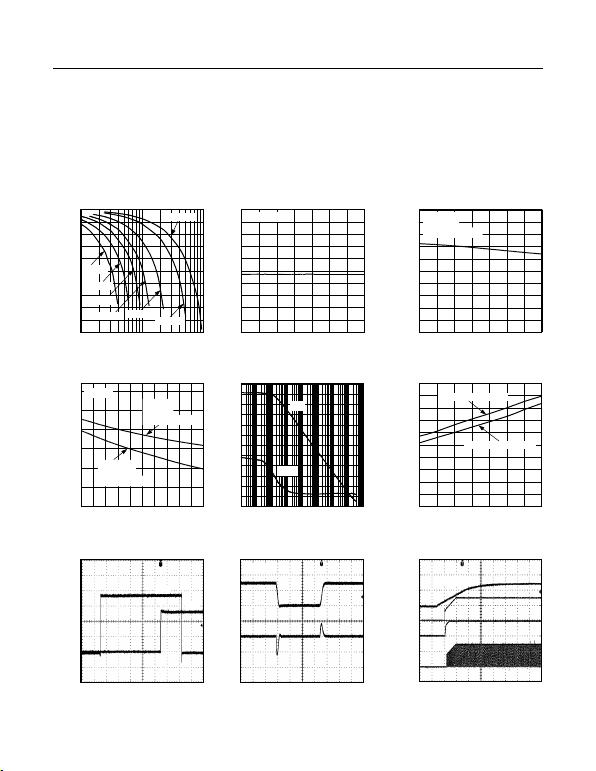VIN = 14V, CIN = 0.1μF, CVCC = 0.1μF // 1μF, CREG5 = 1μF, VON/OFF = 5V, CSS = 0.01μF, CSLOPE = 100pF, RT = 13.7kΩ,
CT = 560pF. TA = +25°C, unless otherwise noted.)
MAX15005 MAXIMUM DUTY CYCLE
vs. OUTPUT FREQUENCY (fOUT)
OUTPUT FREQUENCY (kHz)
MAXIMUM DUTY CYCLE (%)
MAX15004 toc10
50
55
60
65
70
75
80
85
90
95
100
10 100 1000
CT = 220pF
CT = 1500pF
CT = 1000pF CT = 560pF
CT = 2200pF
CT = 3300pF
CT = 100pF
MAX15004 MAXIMUM DUTY CYCLE
vs. TEMPERATURE
TEMPERATURE (°C)
MAXIMUM DUTY CYCLE (%)
MAX15004 toc11
-40 -15 10 35 60 85 110 135
45
46
47
48
49
50
51
52
53
54
55
fOUT = 75kHz
MAX15005 MAXIMUM DUTY CYCLE
vs. TEMPERATURE
TEMPERATURE (°C)
MAXIMUM DUTY CYCLE (%)
MAX15004 toc12
-40 -15 10 35 60 85 110 135
65
67
69
71
73
75
77
79
81
83
85
CT = 560pF
RT = 13.7k
fOSC = fOUT = 150kHz
MAXIMUM DUTY CYCLE
vs. fSYNC/fOSC RATIO
f
SYNC
/f
OSC
RATIO
MAXIMUM DUTY CYCLE (%)
MAX15004 toc13
1.0 1.1 1.2 1.3 1.4 1.5 1.6 1.7 1.8 1.9 2.0
50
55
60
65
70
75
80
CRTCT = 220pF
RRTCT = 10k
fOSC = fOUT = 418kHz
CT = 560pF
RT = 10k
fOSC = fOUT = 180kHz
MAX15005
ERROR AMPLIFIER OPEN-LOOP GAIN
AND PHASE vs. FREQUENCY
FREQUENCY (Hz)
GAIN (dB)
MAX15004 toc14
PHASE (DEGREES)
60
100
140
180
220
260
300
340
-10
0
10
20
30
40
50
60
70
80
90
100
110
0.1 1 10 100 1k 10k 100k 1M 10M
GAIN
PHASE
CS-TO-OUT DELAY vs. TEMPERATURE
TEMPERATURE (°C)
CS-TO-OUT DELAY (ns)
MAX15004 toc15
10
0
20
30
40
50
60
70
80
90
100
-40 -15 10 35 60 85 110 135
VCS OVERDRIVE = 190mV
VCS OVERDRIVE = 50mV
OVI TO OUT DELAY THROUGH
OVERVOLTAGE COMPARATOR
MAX15004 toc16
1µs/div
VOUT
2V/div
VOVI
500mV/div
VOUT
VOVI
DRIVER OUTPUT PEAK SOURCE
AND SINK CURRENT
MAX15004 toc17
400ns/div
VOUT
5V/div
IOUT
1A/div
COUT = 10nF
POWER-UP SEQUENCE THROUGH VIN
MAX15004 toc18
2ms/div
VIN
10V/div
VCC
5V/div
REG5
5V/div
VOUT
5V/div
VON/OFF = 5V
MAX15004A/B-MAX15005A/B 4.5V to 40V Input Automotive
Flyback/Boost/SEPIC
Power-Supply Controllers
Maxim Integrated
7
www.maximintegrated.com
Typical Operating Characteristics (continued)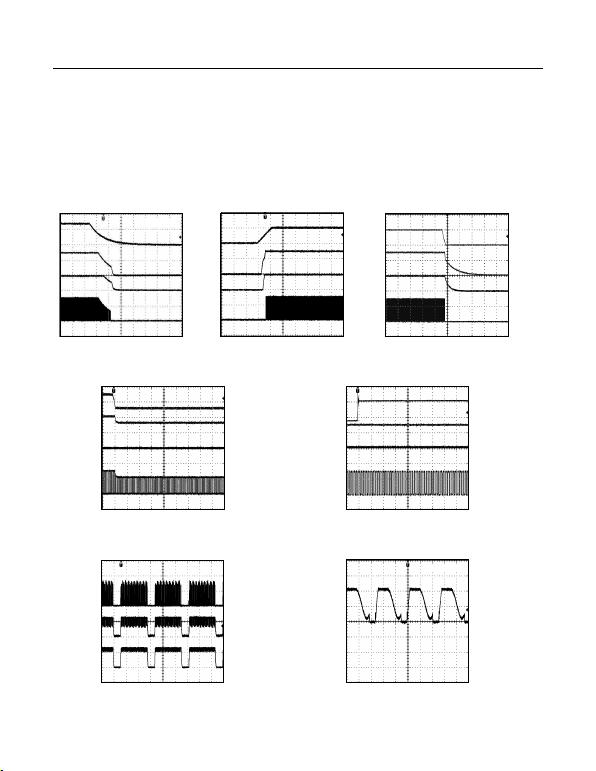VIN = 14V, CIN = 0.1μF, CVCC = 0.1μF // 1μF, CREG5 = 1μF, VON/OFF = 5V, CSS = 0.01μF, CSLOPE = 100pF, RT = 13.7kΩ,
CT = 560pF. TA = +25°C, unless otherwise noted.)
POWER-DOWN SEQUENCE THROUGH VIN
MAX15004 toc19
4ms/div
VIN
10V/div
VCC
5V/div
REG5
5V/div
VOUT
5V/div
VON/OFF = 5V
POWER-UP SEQUENCE
THROUGH ON/OFF
MAX15004 toc20
1ms/div
ON/OFF
5V/div
VCC
5V/div
REG5
5V/div
VOUT
5V/div
POWER-DOWN SEQUENCE
THROUGH ON/OFF
MAX15004 toc21
400ms/div
ON/OFF
5V/div
VCC
5V/div
REG5
5V/div
VOUT
5V/div
LINE TRANSIENT FOR VIN STEP
FROM 14V TO 5.5V
MAX15004 toc22
100µs/div
VIN
10V/div
VCC
5V/div
REG5
5V/div
VOUT
5V/div
LINE TRANSIENT FOR VIN STEP
FROM 14V TO 40V
MAX15004 toc23
100µs/div
VIN
20V/div
VCC
5V/div
REG5
5V/div
VOUT
5V/div
HICCUP MODE FOR FLYBACK CIRCUIT
(FIGURE 7)
MAX15004 toc24
10µs/div
VCS
200mV/div
VANODE
1V/div
ISHORT
500mA/div
DRAIN WAVEFORM IN
FLYBACK CONVERTER (FIGURE 7)
MAX15004 toc25
4µs/div
10V/div
MAX15004A/B-MAX15005A/B 4.5V to 40V Input Automotive
Flyback/Boost/SEPIC
Power-Supply Controllers
Maxim Integrated
8
www.maximintegrated.com
Typical Operating Characteristics (continued)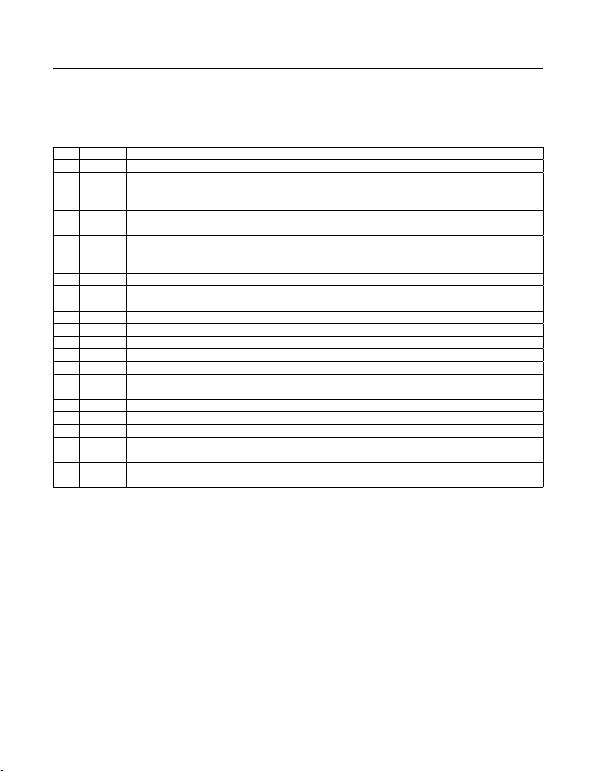PIN NAME FUNCTION
1 IN Input Power Supply. Bypass IN with a minimum 0.1µF ceramic capacitor to PGND.
2 ON/OFF
ON/OFF Input. Connect ON/OFF to IN for always-on operation. To externally program the UVLO threshold of
the IN supply, connect a resistive divider between IN, ON/OFF, and SGND. Pull ON/OFF to SGND to disable the
controller.
3 OVI Overvoltage Comparator Input. Connect a resistive divider between the output of the power supply, OVI, and
SGND to set the output overvoltage threshold.
4 SLOPE
Programmable Slope Compensation Capacitor Input. Connect a capacitor (CSLOPE) to SGND to set the amount
of slope compensation.
Slope compensation = (2.5 x 10-9)/CSLOPE mV/µs with CSLOPE in farads.
5 N.C. No Connection. Not internally connected.
6 RTCT Oscillator-Timing Network Input. Connect a resistor from RTCT to REG5 and a capacitor from RTCT to SGND to
set the oscillator frequency (see the Oscillator Frequency/External Synchronization section).
7 SGND Signal Ground. Connect SGND to SGND plane.
8 SYNC External-Clock Synchronization Input. Connect SYNC to SGND when not using an external clock.
9 SS Soft-Start Capacitor Input. Connect a capacitor from SS to SGND to set the soft-start time interval.
10 FB Internal Error-Amplier Inverting Input. The noninverting input is internally connected to SS.
11 COMP Error-Amplier Output. Connect the frequency compensation network between FB and COMP.
12 CS Current-Sense Input. The current-sense signal is compared to a signal proportional to the error-amplier output
voltage.
13 REG5 5V Low-Dropout Regulator Output. Bypass REG5 with a 1µF ceramic capacitor to SGND.
14 PGND Power Ground. Connect PGND to the power ground plane.
15 OUT Gate Driver Output. Connect OUT to the gate of the external n-channel MOSFET.
16 VCC
7.4V Low-Dropout Regulator Output—Driver Power Source. Bypass VCC with 0.1µF and 1µF or higher ceramic
capacitors to PGND. Do not connect external supply or bootstrap to VCC.
EP Exposed Pad (MAX15004A/MAX15005A only). Connect EP to the SGND plane to improve thermal performance.
Do not use the EP as an electrical connection.
MAX15004A/B-MAX15005A/B 4.5V to 40V Input Automotive
Flyback/Boost/SEPIC
Power-Supply Controllers
www.maximintegrated.com Maxim Integrated
9
Pin Description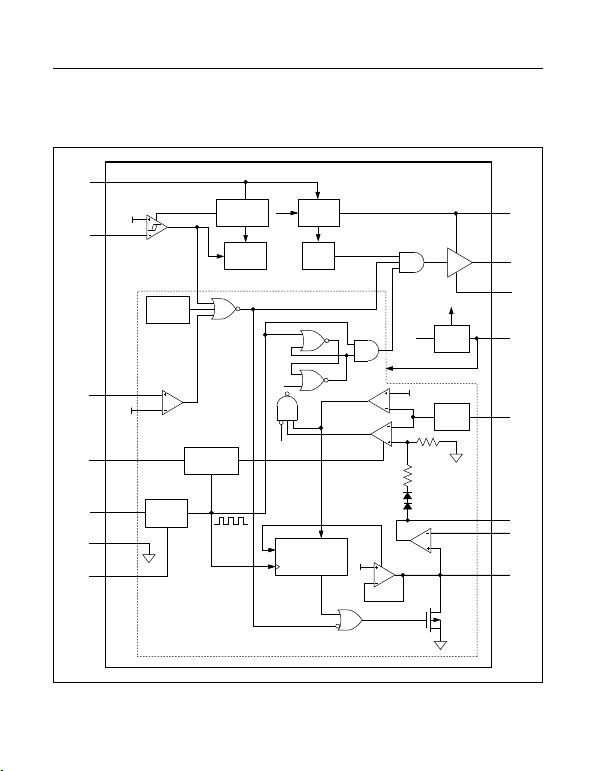MAX15004A/B
MAX15005A/B
PREREGULATOR
REFERENCE
SLOPE
COMPENSATION
EAMP
REF-AMP
THERMAL
SHUTDOWN
OV-COMP
ON/OFF
COMP
OSCILLATOR
7
CONSECUTIVE
EVENTS
COUNTER
7.4V LDO
REG
5V LDO
REG
UVB
VCC
UVB
3.5V
UVLO
50ns
DELAY
DRIVER
R
0.3V
2R
SS_OK
RESET
1.228V
1.228V
1.228V
1
2
3
4
6
7
8
SYNC
SGND
RTCT
SLOPE
OVI
ON/OFF
IN
VCC
16
OUT
15
PGND
14
REG5
13
CS
12
COMP
11
FB
10
SS
9
OFF
OFF
SET
RESET
OVRLD
CLK
OVRLD
PWM-
COMP
ILIMIT
COMP
MAX15004A/B-MAX15005A/B 4.5V to 40V Input Automotive
Flyback/Boost/SEPIC
Power-Supply Controllers
www.maximintegrated.com Maxim Integrated
10
Functional Diagram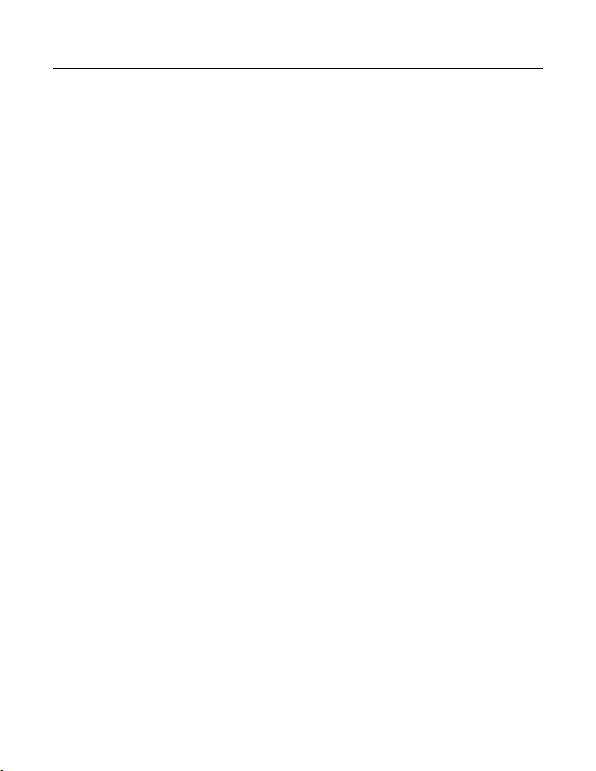Detailed Description
The MAX15004A/B/MAX15005A/B are high-perfor-
mance, current-mode PWM controllers for wide input-
voltage range isolated/nonisolated power supplies. These
controllers are for use as general-purpose boost, flyback,
and SEPIC controllers. The input voltage range of 4.5V
to 40V makes it ideal in automotive applications such as
vacuum fluorescent display (VFD) power supplies. The
internal low-dropout regulator (VCC regulator) enables the
MAX15004A/B/MAX15005A/B to operate directly from an
automotive battery input. The input voltage can go lower
than 4.5V after startup if IN is bootstrapped to a boosted
output voltage.
The undervoltage lockout (ON/OFF) allows the devices
to program the input-supply startup voltage and ensures
predictable operation during brownout conditions.
The devices contain two internal regulators, VCC and
REG5. The VCC regulator output voltage is set at 7.4V
and REG5 regulator output voltage at 5V ±2%. The input
undervoltage lockout (UVLO) circuit monitors the VCC
voltage and turns off the converter when the VCC voltage
drops below 3.5V (typ).
An external resistor and capacitor network programs
the switching frequency from 15kHz to 500kHz. The
MAX15004A/B/MAX15005A/B provide a SYNC input for
synchronization to an external clock. The OUT (FET-driver
output) duty cycle for the MAX15004A/B is 50%. The maxi-
mum duty cycle can be set on MAX15005A/B by selecting
the right combination of RT and CT. The RTCT discharge
current is trimmed to 2%, allowing accurate setting of the
duty cycle for the MAX15005. An internal slope-compen-
sation circuit stabilizes the current loop when operating at
higher duty cycles and can be programmed externally.
The MAX15004/MAX15005 include an internal error
amplifier with 1% accurate reference to regulate the output
in nonisolated topologies using a resistive divider. The
internal reference connected to the noninverting input
of the error amplifier can be increased in a controlled
manner to obtain soft-start. A capacitor connected at SS
to ground programs soft-start to reduce inrush current and
prevent output overshoot.
The MAX15004/MAX15005 include protection features like
hiccup current limit, output overvoltage, and thermal shut-
down. The hiccup current-limit circuit reduces the power
MAX15005 converter during severe fault conditions. The
overvoltage circuit senses the output using the path differ-
ent from the feedback path to provide meaningful overvolt-
age protection. During continuous high input operation, the
power dissipation into the MAX15004/MAX15005 could
exceed its limit. Internal thermal shutdown protection safely
turns off the converter when the junction heats up to 160°C.
Current-Mode Control Loop
The advantages of current-mode control overvoltage-
mode control are twofold. First, there is the feed-forward
characteristic brought on by the controller’s ability to adjust
for variations in the input voltage on a cycle-by-cycle
basis. Secondly, the stability requirements of the current-
mode controller are reduced to that of a single-pole
system unlike the double pole in voltage-mode control.
The MAX15004/MAX15005 offer peak current-mode
control operation to make the power supply easy to design
with. The inherent feed-forward characteristic is useful
especially in an automotive application where the input
voltage changes fast during cold-crank and load dump con-
ditions. While the current-mode architecture offers many
advantages, there are some shortcomings. For higher duty-
cycle and continuous conduction mode operation where
the transformer does not discharge during the off duty
cycle, subharmonic oscillations appear. The MAX15004/
MAX15005 offer programmable slope compensation using
a single capacitor. Another issue is noise due to turn-on
of the primary switch that may cause the premature end
of the on cycle. The current-limit and PWM comparator
inputs have leading-edge blanking. All the shortcomings of
the current-mode control are addressed in the MAX15004/
MAX15005, making it ideal to design for automotive power
conversion applications.
Internal Regulators VCC and REG5
The internal LDO converts the automotive battery voltage
input to a 7.4V output voltage (VCC). The VCC output is
set at 7.4V and operates in a dropout mode at input volt-
ages below 7.5V. The internal LDO is capable of delivering
20mA current, enough to provide power to internal control
circuitry and the gate drive. The regulated VCC keeps the
driver output voltage well below the absolute maximum
gate voltage rating of the MOSFET especially during the
double battery and load dump conditions.
The second 5V LDO regulator from VCC to REG5 provides
power to the internal control circuits. This LDO can also be
used to source 15mA of external load current.
Bypass VCC and REG5 with a parallel combination of 1µF
and 0.1µF low-ESR ceramic capacitors. Additional capaci-
tors (up to 22µF) at VCC can be used although they are
not necessary for proper operation of the MAX15004/
MAX15005.
MAX15004A/B-MAX15005A/B 4.5V to 40V Input Automotive
Flyback/Boost/SEPIC
Power-Supply Controllers
www.maximintegrated.com Maxim Integrated
11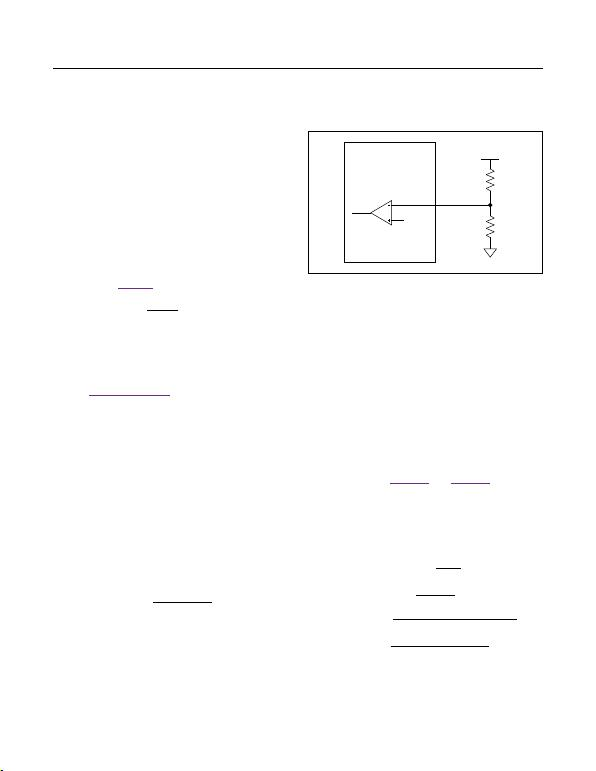Startup Operation/UVLO/ON/OFF
The MAX15004A/B/MAX15005A/B feature two undervolt-
age lockouts (UVLO). The internal UVLO monitors the
VCC-regulator and turns on the converter once VCC rises
above 3.5V. The internal UVLO circuit has about 0.5V
hysteresis to avoid chattering during turn-on.
An external undervoltage lockout can be achieved by
controlling the voltage at the ON/OFF input. The ON/
OFF input threshold is set at 1.23V (rising) with 75mV
hysteresis.
Before any operation can commence, the ON/OFF volt-
age must exceed the 1.23V threshold.
Calculate R1 in Figure 1 by using the following formula:
ON
UVLO
V
R1 1 R2
V

= −×


where VUVLO is the ON/OFF’s 1.23V rising threshold,
and VON is the desired input startup voltage. Choose an
R2 value in the 100kΩ range. The UVLO circuits keep
the PWM comparator, ILIM comparator, oscillator, and
output driver shut down to reduce current consumption
(see the Functional Diagram). The ON/OFF input can be
used to disable the MAX15004/MAX15005 and reduce
the standby current to less than 20μA.
Soft-Start
The MAX15004/MAX15005 are provided with an
externally adjustable soft-start function, saving a number
of external components. The SS is a 1.228V reference
bypass connection for the MAX15004A/B/MAX15005A/B
and also controls the soft-start period. At startup, after
VIN is applied and the UVLO thresholds are reached, the
device enters soft-start. During soft-start, 15μA is sourced
into the capacitor (CSS) connected from SS to GND
causing the reference voltage to ramp up slowly. The
HICCUP mode of operation is disabled during soft-start.
When VSS reaches 1.228V, the output as well as the
HICCUP mode become fully active. Set the soft-start time
(tSS) using following equation:
( )
SS
SS 6
1.23(V) C
t
15 10 A
×
=×
where tSS is in seconds and CSS is in farads.
The soft-start programmability is important to control
the input inrush current issue and also to avoid the
MAX15004/MAX15005 power supply from going into the
unintentional hiccup during the startup. The required soft-
start time depends on the topology used, current-limit
setting, output capacitance, and the load condition.
Oscillator Frequency/External Synchronization
Use an external resistor and capacitor at RTCT to pro-
gram the MAX15004A/B/MAX15005A/B internal oscilla-
tor frequency from 15kHz to 1MHz. The MAX15004A/B
output switching frequency is one-half the programmed
oscillator frequency with a 50% maximum duty-cycle
limit. The MAX15005A/B output switching frequency is
the same as the oscillator frequency. The RC network
connected to RTCT controls both the oscillator frequen-
cy and the maximum duty cycle. The CT capacitor
charges and discharges from (0.1 x VREG5) to (0.55 x
VREG5). It charges through RT and discharges through an
internal trimmed controlled current sink. The maximum
duty cycle is inversely proportional to the discharge time
(tDISCHARGE). See Figure 3a and Figure 3b for a coarse
selection of capacitor values for a given switching frequen-
cy and maximum duty cycle and then use the following
equations to calculate the resistor value to fine-tune the
switching frequency and verify the worst-case maximum
duty cycle.
=
=×
××
=××
=+
MAX
CHARGE OSC
CHARGE
DISCHARGE 3
OSC CHARGE DISCHARGE
D
tf
t
RT 0.7 CT
2.25(V) RT CT
t
(1.33 10 (A) RT) 3.375(V)
1
ftt
where fOSC is the oscillator frequency, RT is the
resistance connected from RTCT to REG5, and CT is
the capacitor connected from RTCT to SGND. For the
Figure 1. Setting the MAX15004A/B/MAX15005A/B
Undervoltage-Lockout Threshold
MAX15004A/B
MAX15005A/B
ON/OFF
R1
1.23V R2
VIN
MAX15004A/B-MAX15005A/B 4.5V to 40V Input Automotive
Flyback/Boost/SEPIC
Power-Supply Controllers
www.maximintegrated.com Maxim Integrated
12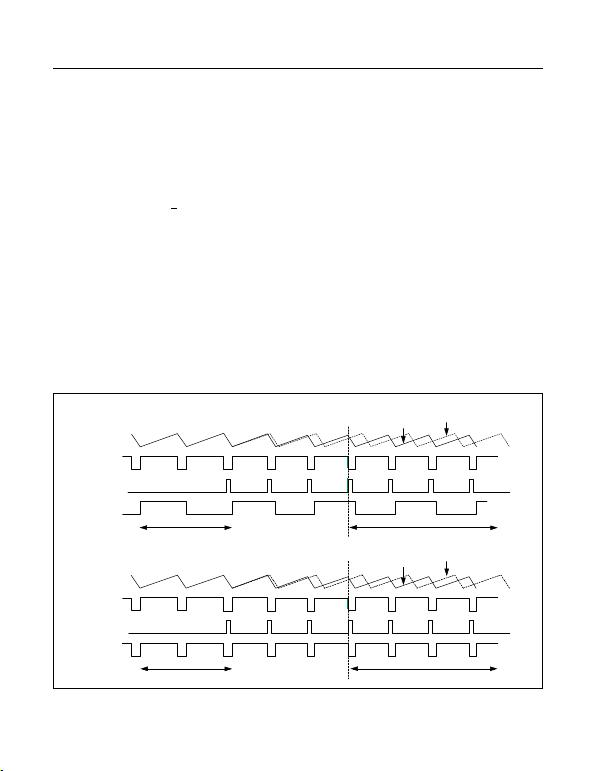most accuracy, CT should include all additional stray
capacitance (typically 25pF to 35pF).
The MAX15004A/B is a 50% maximum duty-cycle part,
while the MAX15005A/B is a 100% maximum duty-cycle
part:
OUT OSC
1
ff
2
=
for the MAX15004A/B and:
OUT OSC
ff=
for the MAX15005A/B.
The MAX15004A/B/MAX15005A/B can be synchronized
using an external clock at the SYNC input. For proper
frequency synchronization, SYNC’s input frequency must
be at least 102% of the programmed internal oscillator
frequency. Connect SYNC to SGND when not using an
external clock. A rising clock edge on SYNC is interpreted
as a synchronization input. If the SYNC signal is lost,
the internal oscillator takes control of the switching rate,
returning the switching frequency to that set by RC net-
work connected to RTCT. This maintains output regulation
even with intermittent SYNC signals.
n-Channel MOSFET Driver
OUT drives the gate of an external n-channel MOSFET.
internally set to approximately 7.4V. The regulated VCC
voltage keeps the OUT voltage below the maximum gate
voltage rating of the external MOSFET. OUT can source
750mA and sink 1000mA peak current. The average
current sourced by OUT depends on the switching
frequency and total gate charge of the external MOSFET.
Error Amplier
The MAX15004A/B/MAX15005A/B include an internal
error amplifier. The noninverting input of the error ampli-
fier is connected to the internal 1.228V reference and
feedback is provided at the inverting input. High 100dB
open-loop gain and 1.6MHz unity-gain bandwidth allow
good closed-loop bandwidth and transient response.
Figure 2. Timing Diagram for Internal Oscillator vs. External SYNC and DMAX Behavior
RTCT
CLKINT
SYNC
OUT
RTCT
CLKINT
SYNC
OUT
D = 50% D = 50%
D = 81.25% D = 80%
WITH SYNC
INPUT
MAX15004A/B (DMAX = 50%)
MAX15005A/B (DMAX = 81%)
WITHOUT
SYNC INPUT
WITH SYNC
INPUT
WITHOUT
SYNC INPUT
MAX15004A/B-MAX15005A/B 4.5V to 40V Input Automotive
Flyback/Boost/SEPIC
Power-Supply Controllers
www.maximintegrated.com Maxim Integrated
13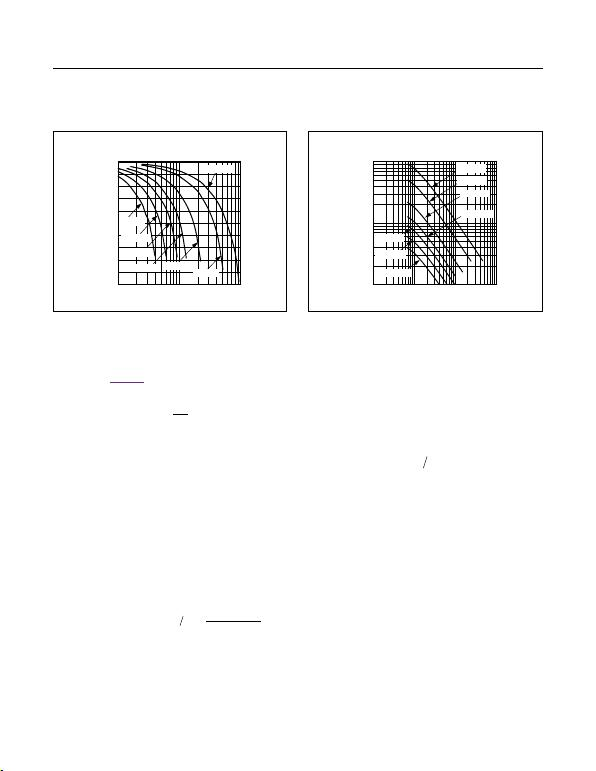Moreover, the source and sink current capability of 2mA
provides fast error correction during the output load
transient. For Figure 5, calculate the power-supply output
voltage using the following equation:
A
OUT REF
B
R
V 1V
R

= +


where VREF = 1.228V. The amplifier’s noninverting input
is internally connected to a soft-start circuit that gradu-
ally increases the reference voltage during startup. This
forces the output voltage to come up in an orderly and
well-defined manner under all load conditions.
Slope Compensation
The MAX15004A/B/MAX15005A/B use an internal ramp
generator for slope compensation. The internal ramp
signal resets at the beginning of each cycle and slews at
the rate programmed by the external capacitor connected
to SLOPE. The amount of slope compensation needed
depends on the downslope of the current waveform.
Adjust the MAX15004A/B/MAX15005A/B slew rate up to
110mV/μs using the following equation:
9
SLOPE
2.5 10 (A)
Slope compensation (mV µs) C
×
=
where CSLOPE is the external capacitor at SLOPE in
Current Limit
The current-sense resistor (RCS), connected between the
source of the MOSFET and ground, sets the current limit.
The CS input has a voltage trip level (VCS) of 305mV. The
current-sense threshold has 5% accuracy. Set the current-
limit threshold 20% higher than the peak switch current at
the rated output power and minimum input voltage. Use
the following equation to calculate the value of RS:
( )
S CS PK
R V I 1.2= ×
where IPRI is the peak current that flows through the
MOSFET at full load and minimum VIN.
When the voltage produced by this current (through the
current-sense resistor) exceeds the current-limit com-
parator threshold, the MOSFET driver (OUT) quickly
terminates the on-cycle. In most cases, a short-time
constant RC filter is required to filter out the leading-edge
spike on the sense waveform. The amplitude and width
of the leading edge depends on the gate capacitance,
drain capacitance (including interwinding capacitance),
and switching speed (MOSFET turn-on time). Set the RC
time constant just long enough to suppress the leading
edge. For a given design, measure the leading spike at
the highest input and rated output load to determine the
value of the RC filter.
Figure 3a. MAX15005 Maximum Duty Cycle vs. Output
Frequency.
Figure 3b. Oscillator Frequency vs. RT/CT
MAX15005 MAXIMUM DUTY CYCLE
vs. OUTPUT FREQUENCY (fOUT)
OUTPUT FREQUENCY (kHz)
MAXIMUM DUTY CYCLE (%)
50
55
60
65
70
75
80
85
90
95
100
10 100 1000
CT = 220pF
CT = 1500pF
CT = 1000pF CT = 560pF
CT = 2200pF
CT = 3300pF
CT = 100pF
OSCILLATOR FREQUENCY (fOSC)
vs. RT/CT
RT (k)
OSCILLATOR FREQUENCY (kHz)
1 10 100 1000
10
100
1000
CT = 220pF
CT = 1500pF
CT = 1000pF
CT = 560pF
CT = 2200pF
CT = 3300pF
CT = 100pF
MAX15004A/B-MAX15005A/B 4.5V to 40V Input Automotive
Flyback/Boost/SEPIC
Power-Supply Controllers
www.maximintegrated.com Maxim Integrated
14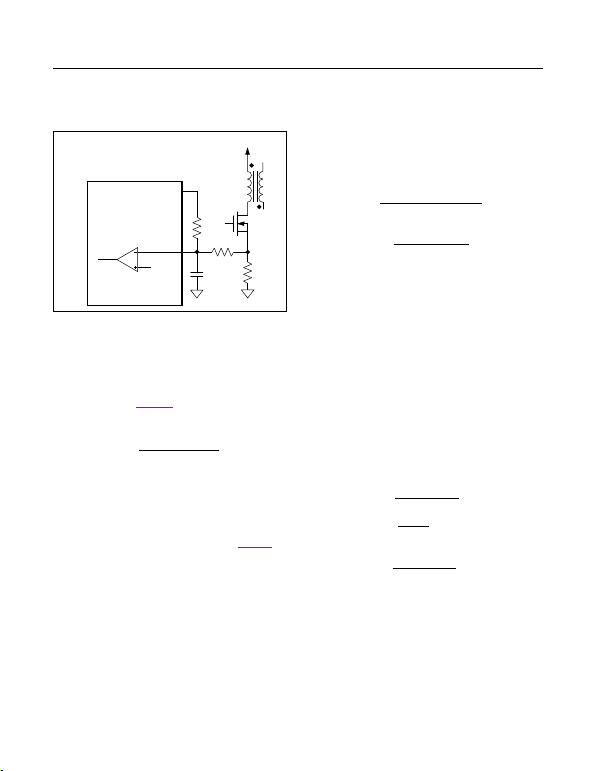The low 305mV current-limit threshold reduces the power
dissipation in the current-sense resistor. The current-limit
threshold can be further reduced by adding a DC offset
to the CS input from REG5 voltage. Do not reduce the
current-limit threshold below 150mV as it may cause
noise issues. See Figure 4. For a new value of the
current-limit threshold (VILIM_LOW), calculate the value of
R1 using the following equation:
CS
ILIM_LOW
4.75 R
R1 0.290 V
×
=
Applications Information
Boost Converter
The MAX15004A/B/MAX15005A/B can be configured for
step-up conversion. The boost converter output can be
fed back to IN through a Schottky diode (see Figure 5) so
the controller can function during low voltage conditions
such as cold-crank. Use a Schottky diode (DVIN) in the
VIN path to avoid backfeeding the input source. Use the
equations in the following sections to calculate inductor
(LMIN), input capacitor (CIN), and output capacitor (COUT)
when using the converter in boost operation.
Inductor Selection in Boost Conguration
Using the following equation, calculate the minimum
inductor value so that the converter remains in continuous
mode operation at minimum output current (IOMIN):
2
IN
MIN OUT OUT OMIN
OUT D IN
OUT D DS
OMIN O O
VD
L 2f V I
where:
V VV
D V VV
and
I (0.1 I ) to (0.25 I )
× ×η
=×× ×
+
=+
=××
The higher value of IOMIN reduces the required
inductance; however, it increases the peak and RMS
currents in the switching MOSFET and inductor. Use
IOMIN from 10% to 25% of the full load current. The VD is
the forward voltage drop of the external Schottky diode,
D is the duty cycle, and VDS is the voltage drop across
the external switch. Select the inductor with low DC
resistance and with a saturation current (ISAT) rating
higher than the peak switch current limit of the converter.
Input Capacitor Selection in Boost
Conguration
The input current for the boost converter is continuous
and the RMS ripple current at the input capacitor is
low. Calculate the minimum input capacitor value and
maximum ESR using the following equations:
L
IN OUT Q
ESR
L
IN DS
LOUT
ID
C 4f V
V
ESR I
where :
(V V ) D
I Lf
∆×
=× ×∆
=
×
∆= ×
VDS is the total voltage drop across the external MOSFET
plus the voltage drop across the inductor ESR. ΔIL is
peak-to-peak inductor ripple current as calculated above.
ΔVQ is the portion of input ripple due to the capacitor
Figure 4. Reducing Current-Sense Threshold
MAX15004A/B-MAX15005A/B 4.5V to 40V Input Automotive
Flyback/Boost/SEPIC
Power-Supply Controllers
www.maximintegrated.com Maxim Integrated
15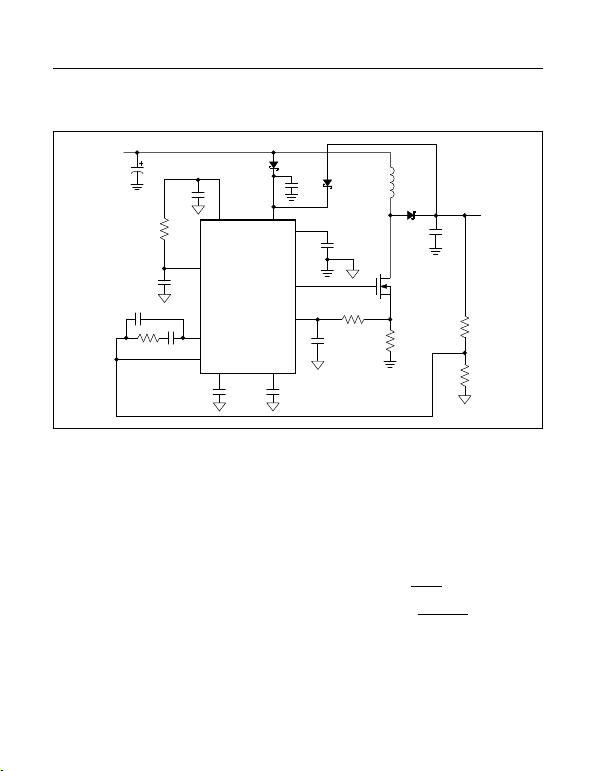discharge and ΔVESR is the contribution due to ESR of
the capacitor. Assume the input capacitor ripple contribu-
tion due to ESR (ΔVESR) and capacitor discharge (ΔVQ)
is equal when using a combination of ceramic and alu-
minum capacitors. During the converter turn-on, a large
current is drawn from the input source especially at high
output to input differential. The MAX15004/MAX15005
are provided with a programmable soft-start; however, a
large storage capacitor at the input may be necessary to
avoid chattering due to finite hysteresis.
Output Capacitor Selection in
Boost Conguration
For the boost converter, the output capacitor supplies the
load current when the main switch is on. The required out-
put capacitance is high, especially at higher duty cycles.
Also, the output capacitor ESR needs to be low enough to
minimize the voltage drop due to the ESR while support-
ing the load current. Use the following equations to calcu-
late the output capacitor, for a specified output ripple. All
ripple values are peak-to-peak.
ESR
O
O MAX
OUT Q OUT
V
ESR I
ID
CVf
=
×
=∆×
IO is the load current, ΔVQ is the portion of the ripple due to
the capacitor discharge, and ΔVESR is the contribution due
to the ESR of the capacitor. DMAX is the maximum duty
cycle at the minimum input voltage. Use a combination
of low-ESR ceramic and high-value, low-cost aluminum
capacitors for lower output ripple and noise.
Figure 5. Application Schematic
MAX15004A/B
MAX15005A/B
IN
CVCC
4.7µF
DVBS
DVIN
RCS
D3
PGND
Q
L
RS
COUT
RB
RA
CVIN
1µF
REG5
SS
SLOPE
11
6
13
16
15
12
1
49
10
RT
CT
VCC
OUT
CS
VOUT
RTCT
COMP
CREG5
0.1µF
VIN
18V
CIN
CCS
CSS
CSLOPE
RF CF
FB
CFF
MAX15004A/B-MAX15005A/B 4.5V to 40V Input Automotive
Flyback/Boost/SEPIC
Power-Supply Controllers
www.maximintegrated.com Maxim Integrated
16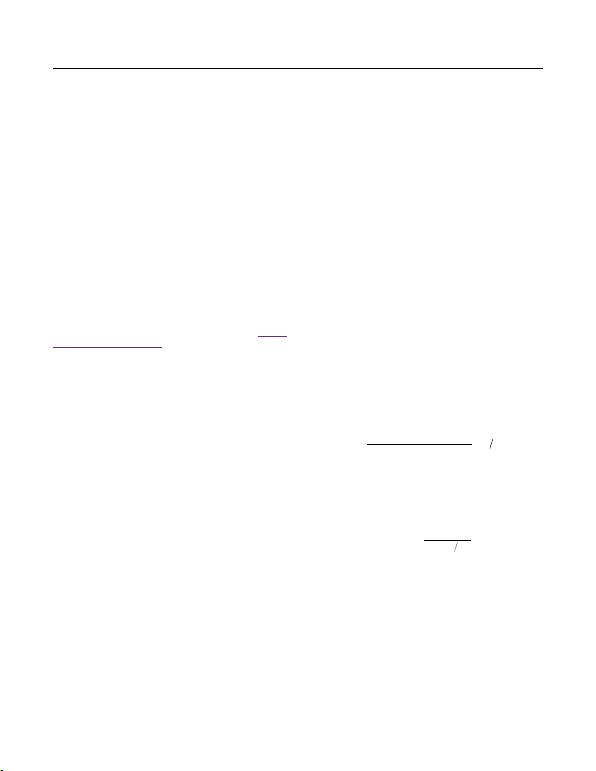Calculating Power Loss in Boost Converter
The MAX15004A/MAX15005A devices are available in
a thermally enhanced package and can dissipate up to
1.7W at +70°C ambient temperature. The total power
dissipation in the package must be limited so that the
junction temperature does not exceed its absolute maxi-
mum rating of +150°C at maximum ambient temperature;
however, Maxim recommends operating the junction at
The average supply current (IDRIVE-GATE) required by
the switch driver is:
DRIVE GATE g OUT
I Qf
= ×
where Qg is total gate charge at 7.4V, a number available
from MOSFET data sheet.
The supply current in the MAX15004A/B/MAX15005A/B
is dependent on the switching frequency. See the Typical
Operating Characteristics to find the supply current
ISUPPLY of the MAX15004A/B/MAX15005A/B at a given
operating frequency. The total power dissipation (PT)
in the device due to supply current (ISUPPLY) and the
current required to drive the switch (IDRIVEGATE) is
calculated using following equation.
T INMAX SUPPLY DRIVE GATE
P V (I I )
=×+
MOSFET Selection in Boost Converter
The MAX15004A/B/MAX15005A/B drive a wide variety
of n-channel power MOSFETs. Since VCC limits the OUT
output peak gate-drive voltage to no more than 11V, a
12V (max) gate voltage-rated MOSFET can be used with-
out an additional clamp. Best performance, especially at
low-input voltages (5VIN), is achieved with low-threshold
n-channel MOSFETs that specify on-resistance with a
gate-source voltage (VGS) of 2.5V or less. When select-
ing the MOSFET, key parameters can include:
1) Total gate charge (Qg).
2) Reverse-transfer capacitance or charge (CRSS).
3) On-resistance (RDS(ON)).
4) Maximum drain-to-source voltage (VDS(MAX)).
5) Maximum gate frequencies threshold voltage
(VTH(MAX)).
At high switching, dynamic characteristics (parameters 1
and 2 of the above list) that predict switching losses have
more impact on efficiency than RDS(ON), which predicts
DC losses. Qg includes all capacitances associated
with charging the gate. The VDS(MAX) of the selected
MOSFET must be greater than the maximum output volt-
age setting plus a diode drop. The 10V additional margin
is recommended for spikes at the MOSFET drain due to
the inductance in the rectifier diode and output capacitor
path. In addition, Qg helps predict the current needed to
drive the gate at the selected operating frequency when
the internal LDO is driving the MOSFET.
Slope Compensation in Boost Conguration
The MAX15004A/B/MAX15005A/B use an internal
ramp generator for slope compensation to stabilize the
current loop when operating at duty cycles above 50%. It is
than 50% duty cycle to improve the noise immunity. The
slope compensations should be optimized because too
much slope compensation can turn the converter into the
voltage-mode control. The amount of slope compensa-
tion required depends on the downslope of the inductor
current when the main switch is off. The inductor downslope
depends on the input to output voltage differential of the
boost converter, inductor value, and the switching frequen-
cy. Theoretically, the compensation slope should be equal
to 50% of the inductor downslope; however, a little higher
Use the following equation to calculate the required
compensating slope (mc) for the boost converter:
( )
3
OUT IN S
(V V ) R 10
mc mV µs
2L
××
=
The internal ramp signal resets at the beginning of each
cycle and slews at the rate programmed by the external
capacitor connected to SLOPE. Adjust the MAX15004A/B/
MAX15005A/B slew rate up to 110mV/μs using the follow-
ing equation:
9
SLOPE
2.5 10
Cmc(mV µs)
×
=
where CSLOPE is the external capacitor at SLOPE in
Flyback Converter
The choice of the conversion topology is the first stage
in power-supply design. The topology selection criteria
include input voltage range, output voltage, peak currents
in the primary and secondary circuits, efficiency, form fac-
tor, and cost.
For an output power of less than 50W and a 1:2 input
voltage range with small form factor requirements, the
flyback topology is the best choice. It uses a minimum
MAX15004A/B-MAX15005A/B 4.5V to 40V Input Automotive
Flyback/Boost/SEPIC
Power-Supply Controllers
www.maximintegrated.com Maxim Integrated
17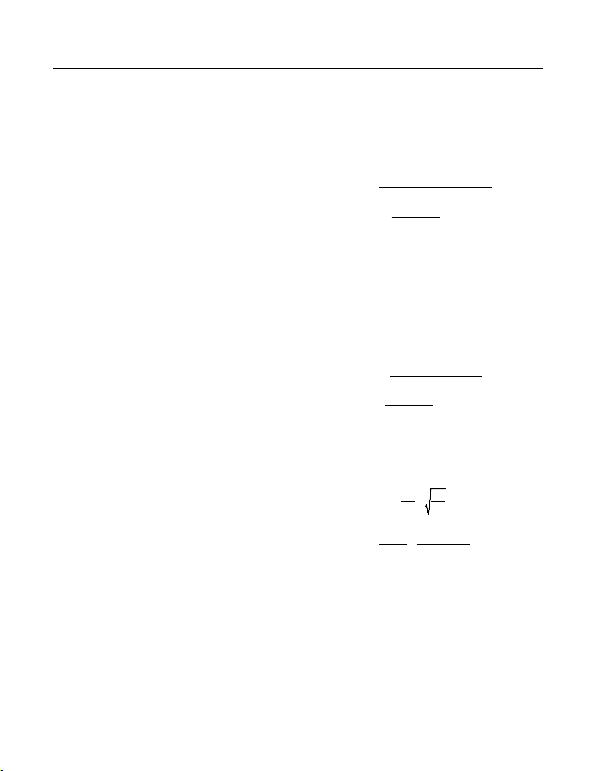of components, thereby reducing cost and form factor.
The flyback converter can be designed to operate either
in continuous or discontinuous mode of operation. In
discontinuous mode of operation, the transformer core
completes its energy transfer during the off-cycle, while
in continuous mode of operation, the next cycle begins
before the energy transfer is complete. The discontinuous
mode of operation is chosen for the present example for
the following reasons:
It maximizes the energy storage in the magnetic com-
ponent, thereby reducing size.
Simplifies the dynamic stability compensation design
(no right-half plane zero).
Higher unity-gain bandwidth.
A major disadvantage of discontinuous mode opera-
tion is the higher peak-to-average current ratio in the
primary and secondary circuits. Higher peak-to-average
current means higher RMS current, and therefore, higher
loss and lower efficiency. For low-power converters, the
advantages of using discontinuous mode easily surpass
the possible disadvantages. Moreover, the drive capabil-
ity of the MAX15004/MAX15005 is good enough to drive
a large switching MOSFET. With the presently available
MOSFETs, power output of up to 50W is easily achievable
with a discontinuous mode flyback topology using the
MAX15004/MAX15005 in automotive applications.
Transformer Design
Step-by-step transformer specification design for a dis-
continuous flyback example is explained below.
Follow the steps below for the discontinuous mode trans-
former:
Step 1) Calculate the secondary winding inductance for
guaranteed core discharge within a minimum off-
time.
Step 2) Calculate primary winding inductance for suf-
ficient energy to support the maximum load.
Step 3) Calculate the secondary and bias winding turns
ratios.
Step 4) Calculate the RMS current in the primary and
estimate the secondary RMS current.
Step 5) Consider proper sequencing of windings and
transformer construction for low leakage.
Step 1) As discussed earlier, the core must be discharged
during the off-cycle for discontinuous mode operation.
The secondary inductance determines the time required
to discharge the core. Use the following equations to
calculate the secondary inductance:
( ) ( )
2
OUT D OFFMIN
SOUT OUT(MAX)
OFF
OFF ON OFF
V VD
L2I f
t
Dtt
××
=+
where:
DOFFMIN = Minimum DOFF
VD = Secondary diode forward voltage drop
IOUT = Maximum output rated current
Step 2) The rising current in the primary builds the energy
stored in the core during on-time, which is then released
to deliver the output power during the off-time. Primary
inductance is then calculated to store enough energy
during the on-time to support the maximum output power.
22
INMIN MAX
POUT OUT(MAX)
ON
ON OFF
VD
L2P f
t
Dtt
× ×η
=××
=+
DMAX = Maximum D.
Step 3) Calculate the secondary to primary turns ratio
(NSP) and the bias winding to primary turns ratio (NBP)
using the following equations:
SS
SP PP
BIAS
BP P OUT
NL
NNL
and
N11.7
NN V 0.35
= =
= = +
The forward bias drops of the secondary diode and the
bias rectifier diode are assumed to be 0.35V and 0.7V,
respectively. Refer to the diode manufacturers data sheet
to verify these numbers.
Step 4) The transformer manufacturer needs the RMS
current maximum values in the primary, secondary,
and bias windings to design the wire diameter for the
different windings. Use only wires with a diameter smaller
MAX15004A/B-MAX15005A/B 4.5V to 40V Input Automotive
Flyback/Boost/SEPIC
Power-Supply Controllers
www.maximintegrated.com Maxim Integrated
18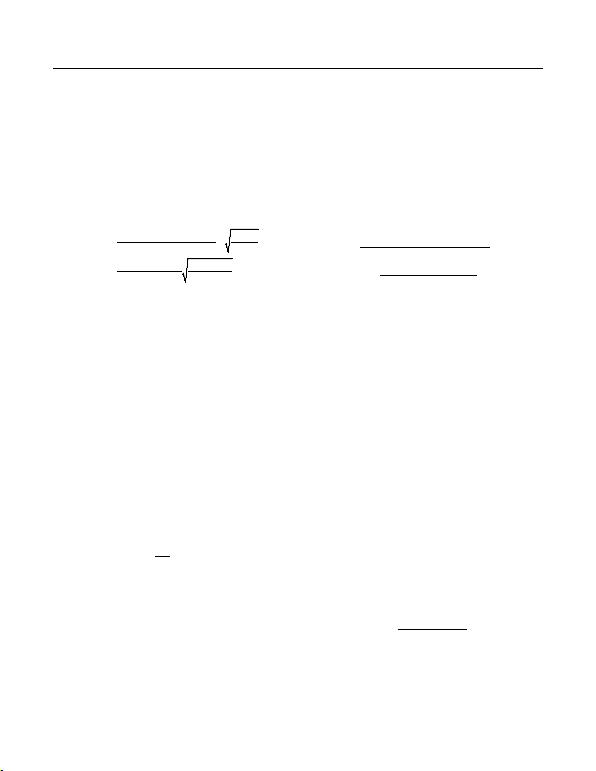than 28AWG to keep skin effect losses under control. To
achieve the required copper cross-section, multiple wires
must be used in parallel. Multifilar windings are common
in high-frequency converters. Maximum RMS currents
in the primary and secondary occur at 50% duty cycle
(minimum input voltage) and maximum output power.
Use the following equations to calculate the primary and
secondary RMS currents:
OUT MAX
PRMS MAX INMIN
OUT OFFMAX
SRMS OFFMAX
PD
I0.5 D V 3
ID
I0.5 D 3
= ×
× ×η×
=×
The bias current for most MAX15004/MAX15005 applica-
tions is about 20mA and the selection of wire depends
more on convenience than on current capacity.
Step 5) The winding technique and the windings sequence
is important to reduce the leakage inductance spike at
switch turn-off. For example, interleave the secondary
between two primary halves. Keep the bias winding close
to the secondary, so that the bias voltage tracks the out-
put voltage.
MOSFET Selection
MOSFET selection criteria include the maximum drain
voltage, peak/RMS current in the primary and the maxi-
mum-allowable power dissipation of the package without
exceeding the junction temperature limits. The voltage
seen by the MOSFET drain is the sum of the input
voltage, the reflected secondary voltage through trans-
former turns ratio and the leakage inductance spike. The
MOSFET’s absolute maximum VDS rating must be higher
than the worst-case (maximum input voltage and output
P
DSMAX INMAX OUT D SPIKE
S
N
V V (V V ) V
N

= + +


Lower maximum VDS requirement means a shorter
channel, lower RDS(ON), lower gate charge, and smaller
package. A lower NP/NS ratio allows a low VDSMAX speci-
fication and keeps the leakage inductance spike under
control. A resistor/diode/capacitor snubber network can
be also used to suppress the leakage inductance spike.
The DC losses in the MOSFET can be calculated using the
value for the primary RMS maximum current. Switching
losses in the MOSFET depend on the operating frequency,
total gate charge, and the transition loss during turn-off.
There are no transition losses during turn-on since the
primary current starts from zero in the discontinuous con-
duction mode. MOSFET derating may be necessary to
avoid damage during system turn-on and any other fault
conditions. Use the following equation to estimate the
power dissipation due to the power MOSFET:
2
MOS DSON PRMS g IN OUTMAX
INMAX PK OFF OUTMAX
2
DS DS OUTMAX
P (1.4 R I ) (Q V f )
V It f
()
4
CV f
2
=× × + ×× +
×× ×
××
+
where:
Qg = Total gate charge of the MOSFET (C) at 7.4V
VIN = Input voltage (V)
tOFF = Turn-off time (s)
CDS = Drain-to-source capacitance (F)
Output Filter Design
The output capacitance requirements for the flyback con-
verter depend on the peak-to-peak ripple acceptable at
during the switch on-time. During the off-cycle, the trans-
former secondary discharges the core replenishing the lost
charge and simultaneously supplies the load current. The
output ripple is the sum of the voltage drop due to charge
loss during the switch on-time and the ESR of the output
capacitor. The high switching frequency of the MAX15004/
MAX15005 reduces the capacitance requirement.
An additional small LC filter may be necessary to
suppress the remaining low-energy high-frequency spikes.
The LC filter also helps attenuate the switching frequency
ripple. Care must be taken to avoid any compensation
problems due to the insertion of the additional LC filter.
Design the LC filter with a corner frequency at more than
a decade higher than the estimated closed-loop, unity-
gain bandwidth to minimize its effect on the phase margin.
Use 1μF to 10μF low-ESR ceramic capacitors and calcu-
late the inductance using following equation:
32
C
1
L
4 10 f C
×××
where fC = estimated converter closed-loop unity-gain
frequency.
MAX15004A/B-MAX15005A/B 4.5V to 40V Input Automotive
Flyback/Boost/SEPIC
Power-Supply Controllers
www.maximintegrated.com Maxim Integrated
19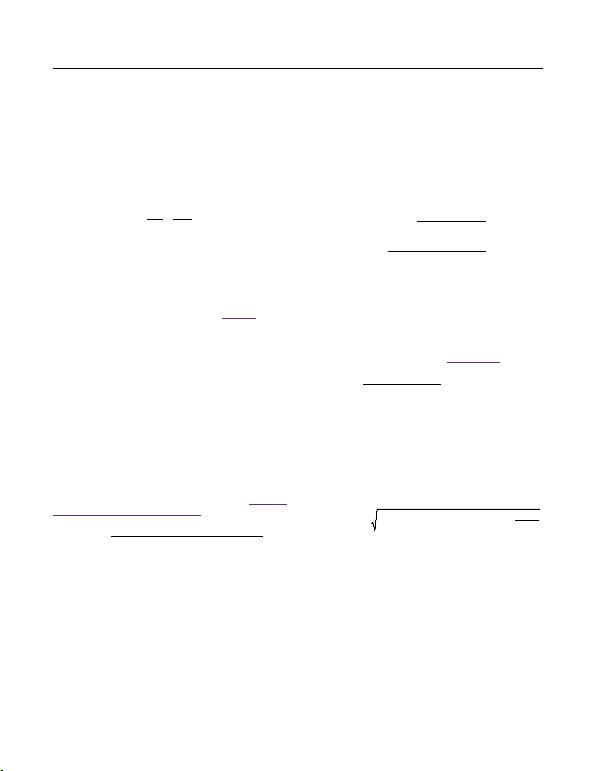SEPIC Converter
The MAX15004A/B/MAX15005A/B can be configured for
SEPIC conversion when the output voltage must be lower
and higher than the input voltage when the input voltage
varies through the operating range. The duty-cycle equa-
tion:
O
IN
VD
V 1D
=
indicates that the output voltage is lower than the input for
a duty cycle lower than 0.5 while VOUT is higher than the
input at a duty cycle higher than 0.5. The inherent advan-
tage of the SEPIC topology over the boost converter is a
complete isolation of the output from the source during a
fault at the output. The SEPIC converter output can be fed
back to IN through a Schottky diode (see Figure 6) so the
controller can function during low voltage conditions such
as cold-crank. Use a Schottky diode (DVIN) in the VIN
path to avoid backfeeding the input source.
a MOSFET, series capacitance, and the rectifier diode.
The inductance is determined by the allowable ripple
current through all the components mentioned above.
Lower ripple current means lower peak and RMS currents
and lower losses. The higher inductance value needed
for a lower ripple current means a larger-sized inductor,
which is a more expensive solution. The inductors (L1 and
L2) can be independent, however, winding them on the
same core reduces the ripple currents.
Calculate the maximum duty cycle using the following
equation and choose the RT and CT values accord-
ingly for a given switching frequency (see the Oscillator
Frequency/External Synchronization section).
OUT D
MAX IN MIN OUT D DS CS
VV
DV V V (V V )

+
=

++ +

where VD is the forward voltage of the Schottky diode,
VCS (0.305V) is the current-sense threshold of the
MAX15004/MAX15005, and VDS is the voltage drop
across the switching MOSFET during the on-time.
Inductor Selection in SEPIC Converter
Use the following equations to calculate the inductance
values. Assume both L1 and L2 are equal and that the
inductor ripple current (ΔIL) is equal to 20% of the input
current at nominal input voltage to calculate the induc-
tance value.
IN MIN MAX
1OUT L
OUT MAX MAX
LMAX
VD
L L L2 2f I
0.2 I D
I(1 D )

×
= = =

× ×∆


××
∆=

×η

where fOUT is the converter switching frequency and
η is the targeted system efficiency. Use the coupled
inductors MSD-series from Coilcraft or PF0553-series from
Pulse Engineering, Inc. Make sure the inductor saturating
current rating (ISAT) is 30% higher than the peak inductor
current calculated using the following equation. Use the
current-sense resistor calculated based on the ILPK value
from the equation below (see the Current Limit section).
OUT MAX MAX
LPK OUT MAX L
MAX
ID
I II
(1 D )

×
= + +∆

×η

MOSFET, Diode, and Series Capacitor
Selection in a SEPIC Converter
For the SEPIC configuration, choose an n-channel
MOSFET with a VDS rating at least 20% higher than the
sum of the output and input voltages. When operating at
a high switching frequency, the gate charge and switch-
ing losses become significant. Use low gate-charge
MOSFETs. The RMS current of the MOSFET is:
22 MAX
MOS RMS LPK LDC LPK LDC
D
I (A) (I ) (I ) (I I ) 3

= + ×


where ILDC = (ILPK - ΔIL).
Use Schottky diodes for higher conversion efficiency. The
reverse voltage rating of the Schottky diode must be high-
er than the sum of the maximum input voltage (VIN-MAX)
and the output voltage. Since the average current flowing
through the diode is equal to the output current, choose
the diode with forward current rating of IOUT-MAX. The
MAX15004A/B-MAX15005A/B 4.5V to 40V Input Automotive
Flyback/Boost/SEPIC
Power-Supply Controllers
www.maximintegrated.com Maxim Integrated
20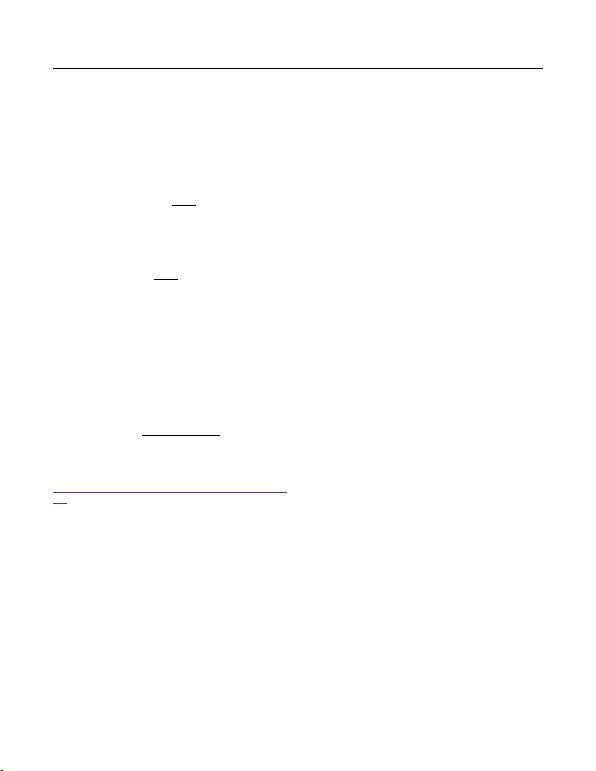current sense (RCS) can be calculated using the current-
limit threshold (0.305V) of MAX15004/MAX15005 and
ILPK. Use a diode with a forward current rating more than
the maximum output current limit if the SEPIC converter
needs to be output short-circuit protected.
CS LPK
0.305
RI
=
Select RCS 20% below the value calculated above.
Calculate the output current limit using the following
equation:
( )
OUT LIM LPK L
D
I II
(1 D)

=×∆


where D is the duty cycle at the highest input voltage
(VIN-MAX).
The series capacitor should be chosen for minimum ripple
voltage (ΔVCP) across the capacitor. We recommend
using a maximum ripple ΔVCP to be 5% of the minimum
input voltage (VIN-MIN) when operating at the minimum
input voltage. The multilayer ceramic capacitor X5R and
X7R series are recommended due to their high ripple
current capability and low ESR. Use the following
equation to calculate the series capacitor CP value.
OUT MAX MAX
CP OUT
ID
CP Vf

×
=

∆×

where ΔVCP is 0.05 x VIN-MIN.
For a further discussion of SEPIC converters, go to
http://pdfserv.maximintegrated.com/en/an/AN1051.
pdf.
Power Dissipation
The MAX15004/MAX15005 maximum power dissipation
depends on the thermal resistance from the die to the
ambient environment and the ambient temperature. The
thermal resistance depends on the device package, PCB
copper area, other thermal mass, and airflow.
Calculate the temperature rise of the die using following
equation:
TJ = TC + (PT x θJC)
or:
TJ = TA + (PT x θJA)
where θJC is the junction-to-case thermal impedance
(3°C/W) of the 16-pin TSSOP-EP package and PT is
power dissipated in the device. Solder the exposed
heat through the board surface, minimizing the case-to-
ambient thermal impedance. Measure the temperature of
the copper area near the device (TC) at worst-case condi-
tion of power dissipation and use 3°C/W as θJC thermal
impedance. The case-to-ambient thermal impedance
JA) is dependent on how well the heat is transferred
from the PCB to the ambient. Use a large copper area
to keep the PCB temperature low. The θJA is 38°C/W for
TSSOP-16-EP and 90°C/W for TSSOP-16 package with
the condition specified by the JEDEC51 standard for a
multilayer board.
MAX15004A/B-MAX15005A/B 4.5V to 40V Input Automotive
Flyback/Boost/SEPIC
Power-Supply Controllers
www.maximintegrated.com Maxim Integrated
21